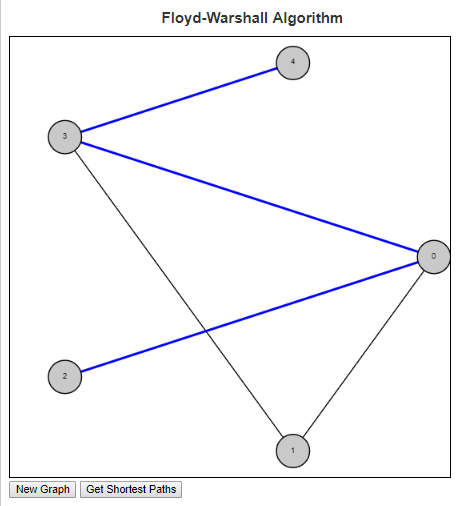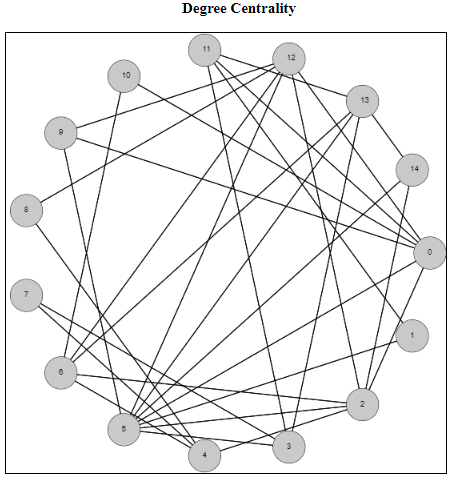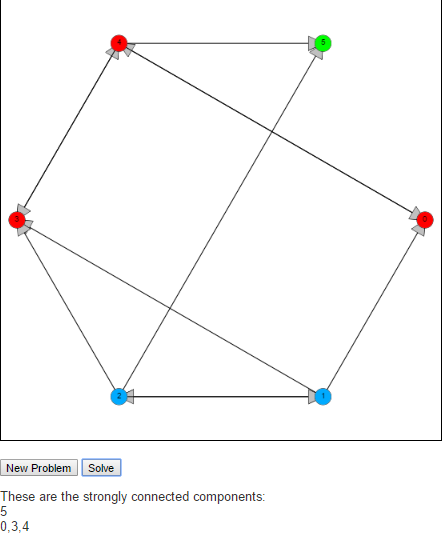# Set Partition Problems

This is my first post of 2019 and my first post in a while. There was one posted a few months ago, but not really geared towards the algorithms and learning focus of the site. I have been doing a lot of coding in my spare time, but honestly life has just gotten in the way. Its not a bad thing, but life is life and sometimes I have to prioritize the things. In particular, I have been having ideas and actually coding things up but the time it takes to clean up code, write a blog entry and finding a nice way to visualize these things has been something that I haven’t been able to really focus on as much as I’ve wanted to.

That said, I want to talk to you about the Set Partition Problem today. You can go to Wikipedia to get more information about this problem, but I will give you a brief introduction to it and then talk about two different approaches to it. The problem assumes that we are given as input a (multi)-set S. The reason we say it is a multi-set and not a simple set is because we can have the same element appear multiple times in the set. So if S1 = {2} and S2 = {2, 2}, then although as sets they are both equal to the set {2} = S1, as multi-sets allow for multiple instances of an element. The elements of S are assumed to be positive integers.

So given this multi-set S, we ask the question of can the elements of S be divided into two smaller multi-sets, C1 and C2 where

• C1 [union] C2 = S
• C1 [intersect] C2 = [empty set]
• [Sigma]_[x in C1] = [Sigma]_[x in C2].C1 [union] C2 = S, C1 [intersect] C2 = [empty set], and [Sigma]_[x in C1] = [Sigma]_[x in C2]

The first two bullets above say that the sets C1 and C2 form a partition of S. The third bullet says that the sums of the elements in the two children multi-sets are equal.

This problem is known to be NP Complete. This means that it is one of the more difficult decision problems. Because of this finding an algorithm that solves this problem exactly will generally take a long running time. And finding an algorithm that runs quickly will more than likely be incorrect in some instances.

I will show you two approaches to this problem. One is based on Dynamic Programming (DP) and one is based on Greedy Algorithms. The DP version solves the problem exactly but has a slow running time. The greedy algorithm is fast but is not guaranteed to always give the correct answer.

Dynamic Programming is based on the principle of optimality. This says that in order to have a correct solution to the overall problem, we need optimal solutions to all subproblems of this problem. This is done by keeping track of a table which can be used for looking up these subproblems and the optimal solutions to these subproblems.

For this problem, we will build a table where the rows represent the final sums and columns represent subsets of the given multi-set (containing the first 0…n elements). The question we are repeatedly asking is “can we find a subset of the set in this column whose sum is exactly the given rowsum?” Here is an example of the DP algorithm on the multi-set {5, 6, 5, 6, 7}.

Greedy Algorithms are generally based on sorting the elements based on some principle and using that to try to answer the underlying question. The main problem with this approach is that it is very short sided because they do not look at the overall picture. Such algorithms are known for finding local optima that are not always globally optimal. The benefit to these problems though is that they are generally easier to code and easier to understand.

For the Set Partition Problem , the Greedy approach is to sort elements in descending order. Once this is done, the goal is to keep two subsets while iterating through the array, adding the element to the smaller of the two sets whenever possible.

For more examples, check out Set Partition Problems

# Binary Puzzles

As you can probably tell, I’m a big fan of puzzles. On one hand you can say that a good puzzle is nothing but particular instance of a complex problem that we’re being asked to solve. What exactly makes a problem complex though?

To a large extent that depends on the person playing the puzzles. Different puzzles are based on different concepts and meant to highlight different concepts. Some puzzles really focus on dynamic programming like the Triangle Sum Puzzles or the Unidirectional TSP Puzzles.

Other puzzles are based on more complicated problems, in many cases instances of NP-complete problems. Unlike the puzzles mentioned above, there is generally no known optimal strategy for solving these puzzles quickly. Some basic examples of these are ones like Independent Set Puzzles, which just give a random (small) instance of the problem and ask users to solve it. Most approaches involve simply using logical deduction to reduce the number of possible choices until a “guess” must be made and then implementing some form of backtracking solution (which is not guessing since you can form a logical conclusion that if the guess you made were true, you reach either (a) a violation of the rules or (b) a completed puzzle).

One day a few months back i was driving home from work and traffic was so bad that i decided to stop at the store. While browsing the books, I noticed a puzzle collection. Among the puzzles I found in that book were the Range Puzzles I posted about earlier. However I also found binary puzzles.

Filled Binary puzzles are based on three simple rules
1. No the adjacent cells in any row or column can contain the same value (so no 000 or 111 in any row or column).
2. Every row must have the same number of zeros and ones.
3. Each row and column must be unique.

There is a paper from 2013 stating that Binary Puzzles are NP Complete. There is another paper that discusses strategies involved in Solving a Binary Puzzle

Once I finished the puzzles in that book the question quickly became (as it always does) where can I get more. I began writing a generator for these puzzles and finished it earlier this year. Now i want to share it with you. You can visit the examples section to play those games at Binary Puzzles.

Below I will go over a sample puzzle and how I go about solving it. First lets look at a 6 by 6 puzzle with some hints given:

 0 1 0 1 0 1 1 0 1 0 0 1 1 0

We look at this table and can first look for locations where we have a “forced move”. An obvious choice for these moves wold be three adjacent cells in the same row or column where two have the same value. A second choice is that when we see that a row or column has the correct number of zeros or ones, the remaining cells in that row or column must have the opposite value.

So in the above puzzle, we can see that the value in cells (2, 2) and (2, 5) must also be a 0 because cells (2, 3) and (2, 4) are both 1. Now we see that column 2 has 5 of its 6 necessary values, and three 0’s. So the last value in this column (2, 6) must be a 1 in order for there to be an equal number of 0s and 1s.

For some easier puzzles these first two move types will get you far enough to completely fill in all the cells. For more advanced puzzles though, this may require a little more thorough analysis.

As always, check it out and let me know what you think.

# Floyd-Warshall Shortest PathsThe Floyd Warshall algorithm is an all pairs shortest paths algorithm. This can be contrasted with algorithms like Dijkstra’s which give the shortest paths from a single node to all other nodes in the graph.

Floyd Warshall’s algorithm works by considering first the edge set of the graph. This is the set of all paths of the graph through one edge. Node pairs that are connected to one another through an edge will have their shortest path set to the length of that edge, while all other node pairs will have their shortest path set to infinity. The program then runs through every triplet of nodes (i, j, k) and checks if the path from i to k and the path from k to j is shorter than the current path from i to j. If so, then the distance and the path is updated.

So lets consider an example on the graph in the image above. The edge set of this graph is E = {(0, 1), (0, 2), (0, 3), (1, 3), (3, 4)}. So our initial table is:

 0 1 2 3 4 0 inf (0, 1) (0, 2) (0, 3) inf 1 (0, 1) inf inf (1, 3) inf 2 (0, 2) inf inf inf inf 3 (0, 3) (1, 3) inf inf (3, 4) 4 inf inf inf (3, 4) inf

As we look to update the paths, we first look for routes that go through node 0:

Because node 0 connects to both node 1 and node 2, but node 1 does not connect to node 2, we have the following truth holding in the matrix above:
cost(0, 1) + cost(0, 2) < cost(1, 2), so we can update the shortest path from node 1 to node 2 to be (1, 0, 2).

Because node 0 connects to both node 2 and node 3, but node 2 does not connect to node 3, we have the following truth holding in the matrix above:
cost(0, 2) + cost(0, 3) < cost(2, 3), so we can update the shortest path from node 2 to node 3 to be (2, 0, 3).

Because node 3 connects to both node 0 and node 4, but node 0 does not connect to node 4, we have the following truth holding in the matrix above:
cost(0, 3) + cost(3, 4) < cost(0, 4), so we can update the shortest path from node 0 to node 4 to be (0, 3, 4).

Because node 3 connects to both node 1 and node 4, but node 1 does not connect to node 4, we have the following truth holding in the matrix above:
cost(1, 3) + cost(3, 4) < cost(1, 4), so we can update the shortest path from node 1 to node 4 to be (1, 3, 4).

Because node 3 connects to both node 2 and node 4, but node 2 does not connect to node 4, we have the following truth now holding:
cost(2, 3) + cost(3, 4) < cost(2, 4), so we can update the shortest path from node 2 to node 4 to be (2, 0, 3, 4).

The final table giving the list of shortest paths from every node to every other node is given below.

 0 1 2 3 4 0 inf (0, 1) (0, 2) (0, 3) (0, 3, 4) 1 (0, 1) inf (1, 0, 2) (1, 3) (1, 3, 4) 2 (0, 2) (1, 0, 2) inf (2, 0, 3) (2, 0, 3, 4) 3 (0, 3) (1, 3) (2, 0, 3) inf (3, 4) 4 (0, 3, 4) (1, 3, 4) (2, 0, 3, 4) (3, 4) inf

To see more examples and to help answer questions, check out the script in my examples section on the Floyd-Warshall algorithm

# Degree Centrality of a GraphI wanted to spend some time on centrality measures of a graph. These are measurements of how important each node (or edge) is to the overall graph. But how do we define, or determine, importance? There is no unique way to answer this question, so there are varying metrics for measuring centrality. Which one you choose depends on several factors including how many other nodes of the graph are included, as well as the run time of the metrics you’re considering.

I have just published a script focusing on the degree centrality metric. The degree centrality metric is called a “walk metric” because it determines how important a node is by how many other nodes that can be reached by walks of up to a certain length. Lets look at the definition of the degree of a node to see if we can understand why it is called a walk metric.

In an undirected graph G = (V, E), the degree of a node u [in] V is the |{v | (u, v) [in] E}|. This is the size of the set of nodes that are connected to node u via a single edge. Another way of describing a single edge is a walk of length one. So the degree metric measures the importance of a node by the number of unique walks of length one.

The normalized degree centrality of a node v in a graph G = (V,E) measures how many nodes are connected to the node v, compared to the maximum possible number of edges that can be connected to this node. Because we are dealing with simple undirected graphs (at most a single edge between any two distinct vertices), this maximum possible number will always be |V – 1|. So the normalized degree can be calculated by dividing the degree of the node (the number of nodes it is connected to) by |V – 1|.

So for the example above, the node 0 has degree 6 because it is connected to nodes 2, 5, 9, 10, 11, and 12. There are 15 total nodes in this graph, so to calculate the normalized degree centrality of the node 0, it will be 6 / 14, which rounds to 0.428571.

To see more examples and to help answer questions, check out the script in my examples section on degree centrality

# Tarjan’s Strongly Connected Components AlgorithmA strongly connected component of a graph is a subgraph S of G where every pair of nodes, u and v in S there is a path from u to v and a path from v to u.

To find these strongly connected components we implement Tarjan’s algorithm. The idea behind Tarjan’s algorithm is to begin by running a depth first search from an arbitrary node in the graph, labeling nodes reachable from this start node in the order they are reached. The algorithm is also interested in the “oldest” node that could be reached by a given node. This is indicated by the keeping track of the lowest label that can be reached from that node. We will call the first property label(v) and the second lowlink(v).

When the algorithm starts label(v) is the same as lowlink(v) whenever a node is discovered. As the algorithm is executed, the DFS is being run on each discovered node, which in turn updates the lowlink(v) property telling of (older) nodes that can be reached. If an older node can be reached, then we update lowlink. If we reach a node that cannot connect to any older nodes after the DFS call, i.e if label(v) is the same a lowlink(v), then this means that this node does not have a path to any node with a lower label. So this node will be the first node of a new strongly connected component.

Feel free to check it out an let me know what you think in the comments below.